# RS Aggarwal Solutions for Class 6 Maths Chapter 8 Algebraic Expressions

## Class 6 RS Aggarwal Chapter 8 – Algebraic Expressions

RS Aggarwal Solutions for Class 6 Maths, Chapter 8 Algebraic Expressions are given here. Students who wish to prepare thoroughly for the exam are advised to go through the RS Aggarwal Solutions for Class 6 Maths Chapter 8 to score good marks.These Solutions need a lot of practice to gain high marks in examinations. By referring RS Aggarwal Solutions, students will understand the Chapter in detail. Practising textbook questions will increase the confidence of the students and help to understand the topics which are talked about, in this Chapter.

Students can go through the RS Aggarwal Solutions for Class 6 Chapter 8 Maths which have been solved by BYJU’S experts in pdf format. Download pdf of Class 6 Chapter 8 in their respective links.

## Download PDF of RS Aggarwal Solutions for Class 6 Maths Chapter 8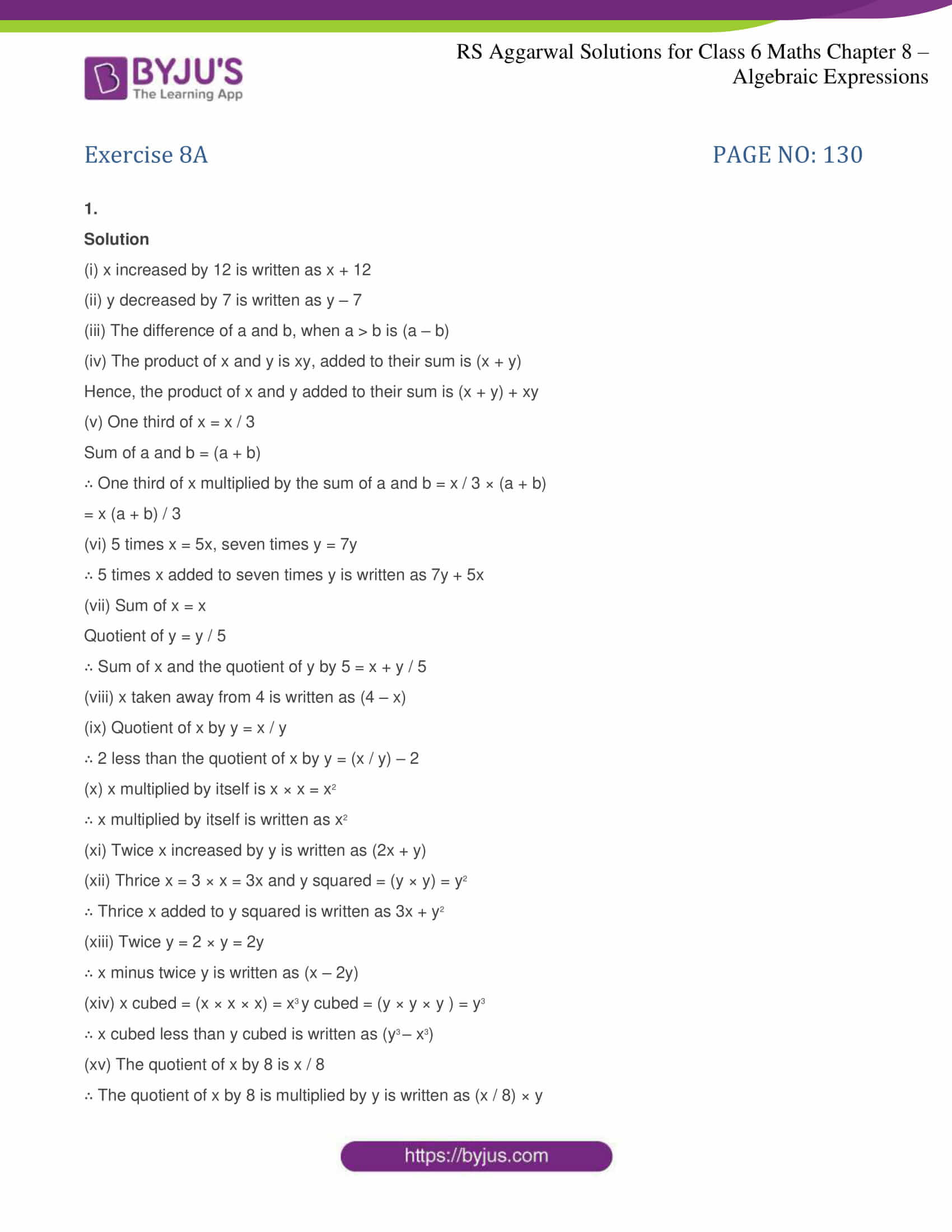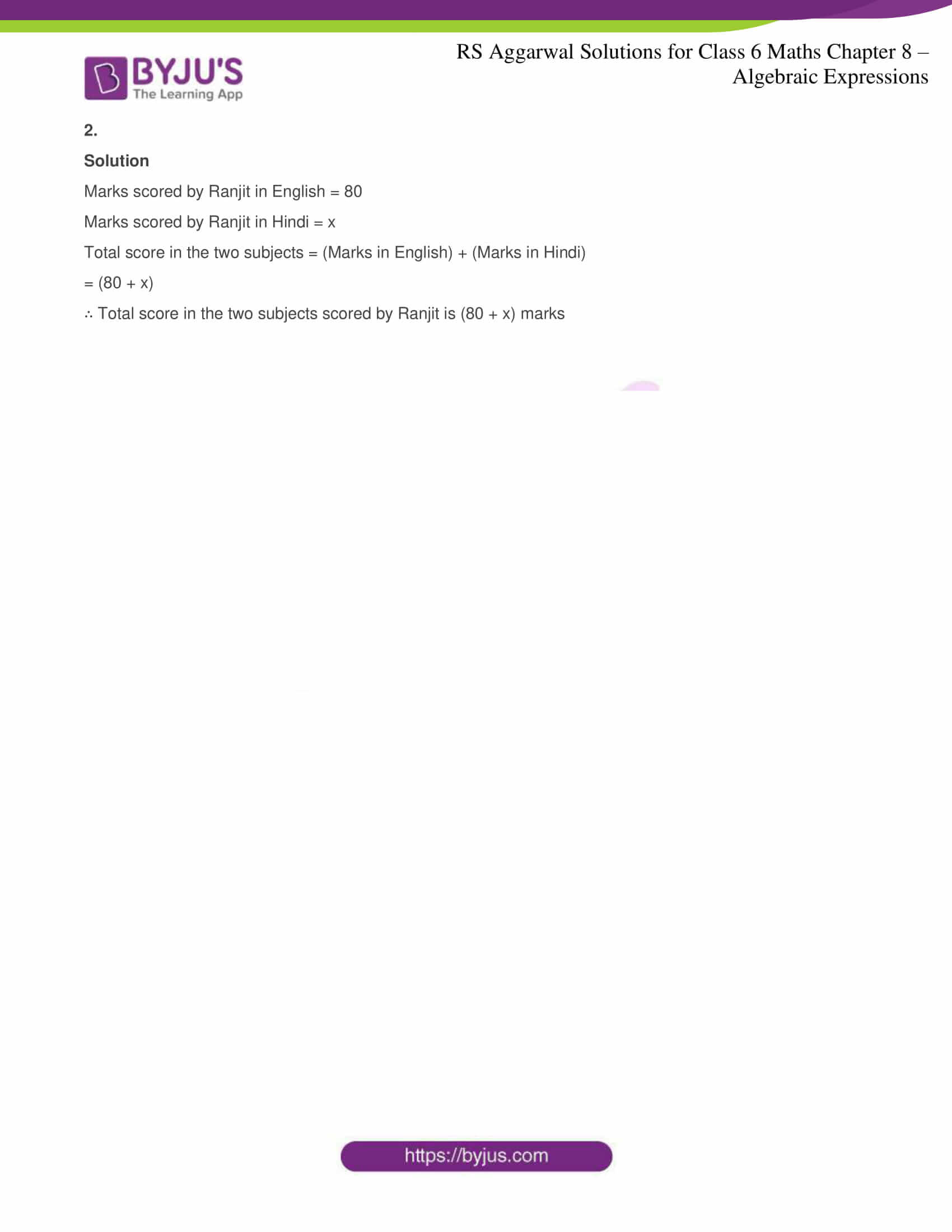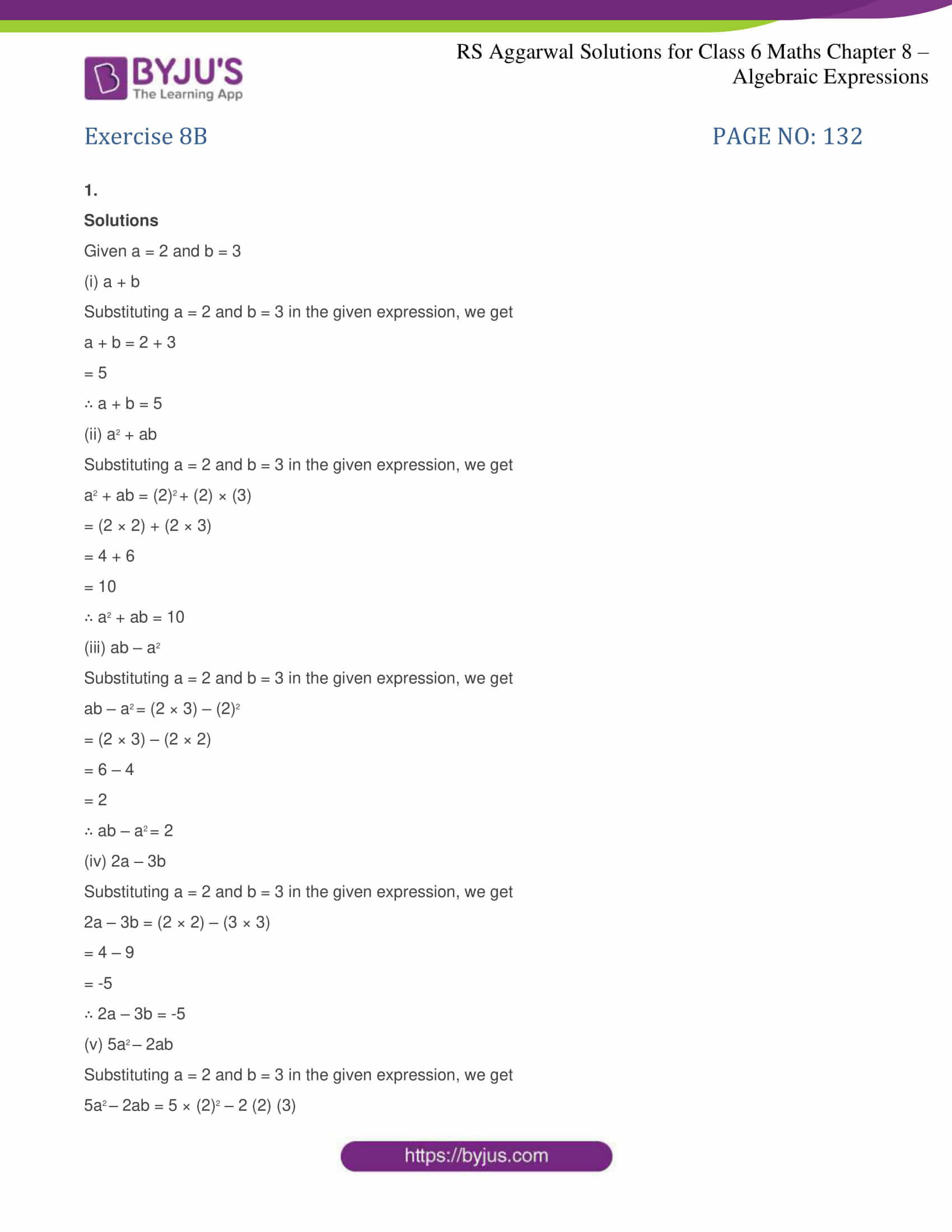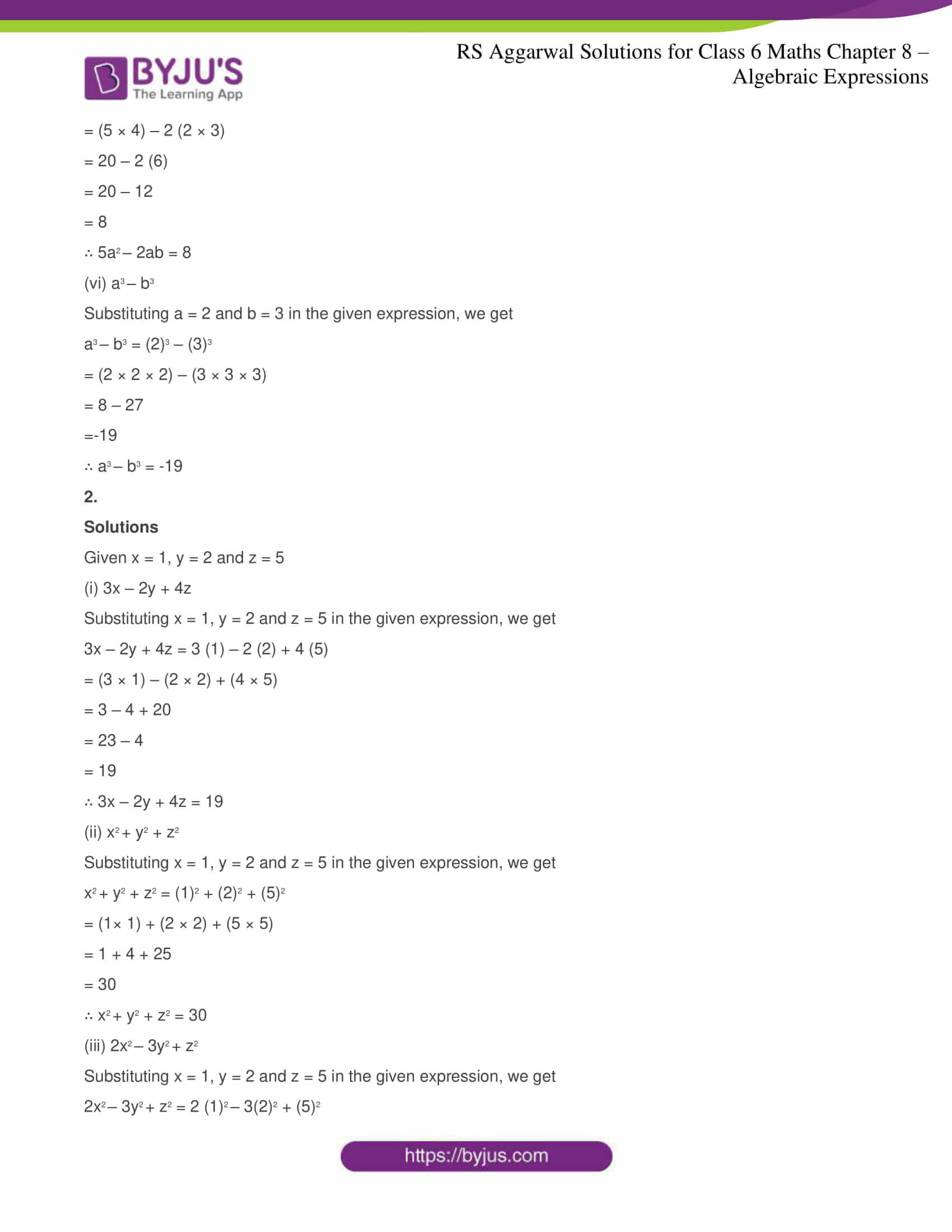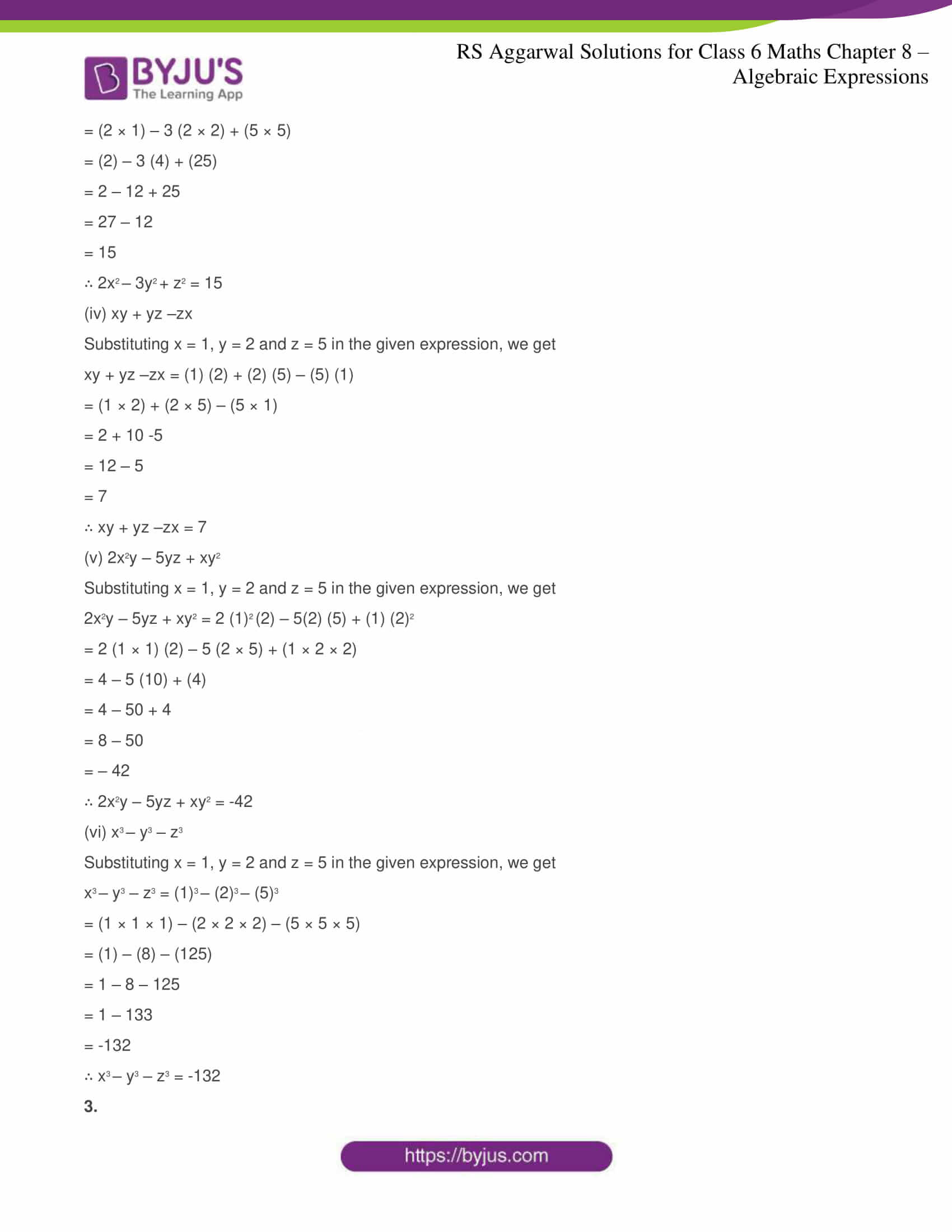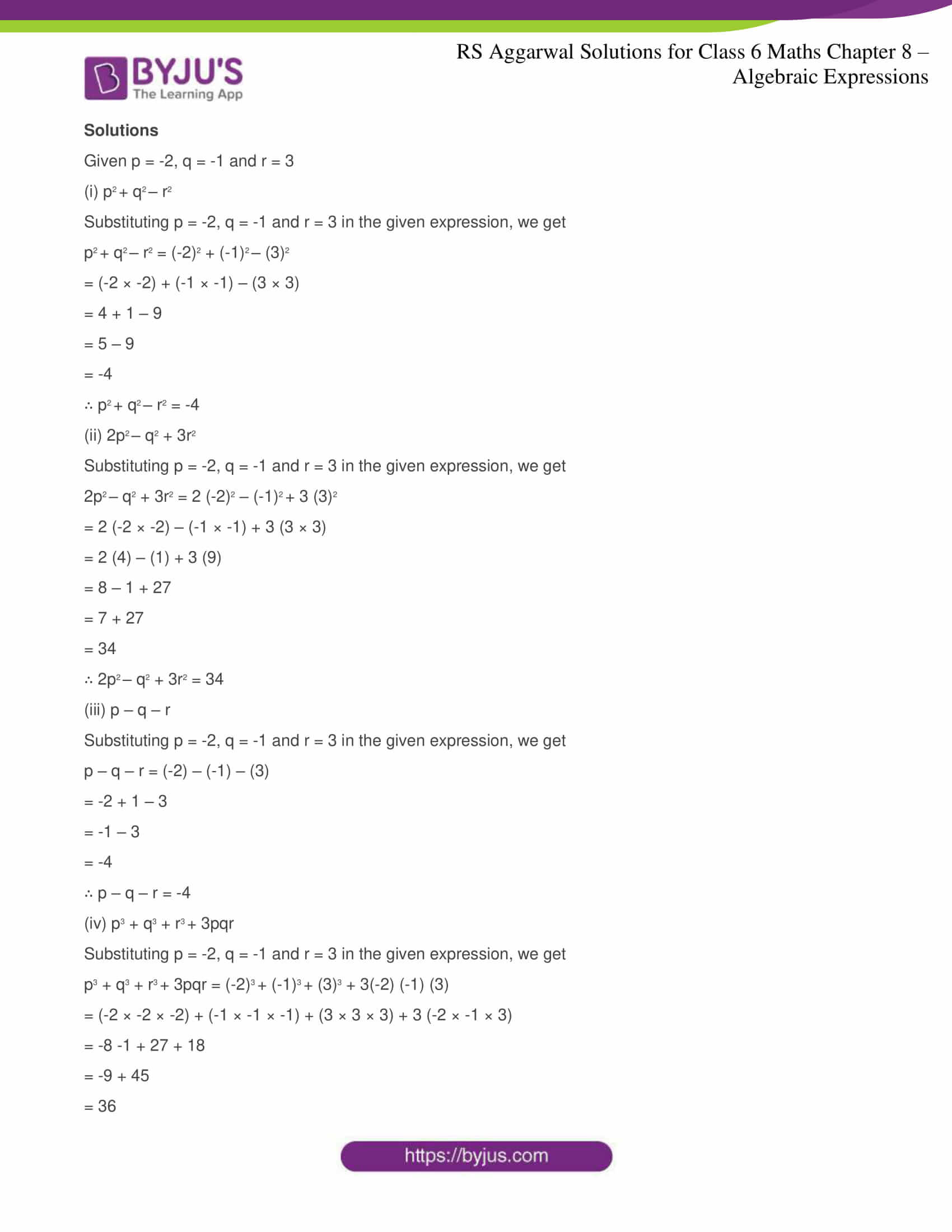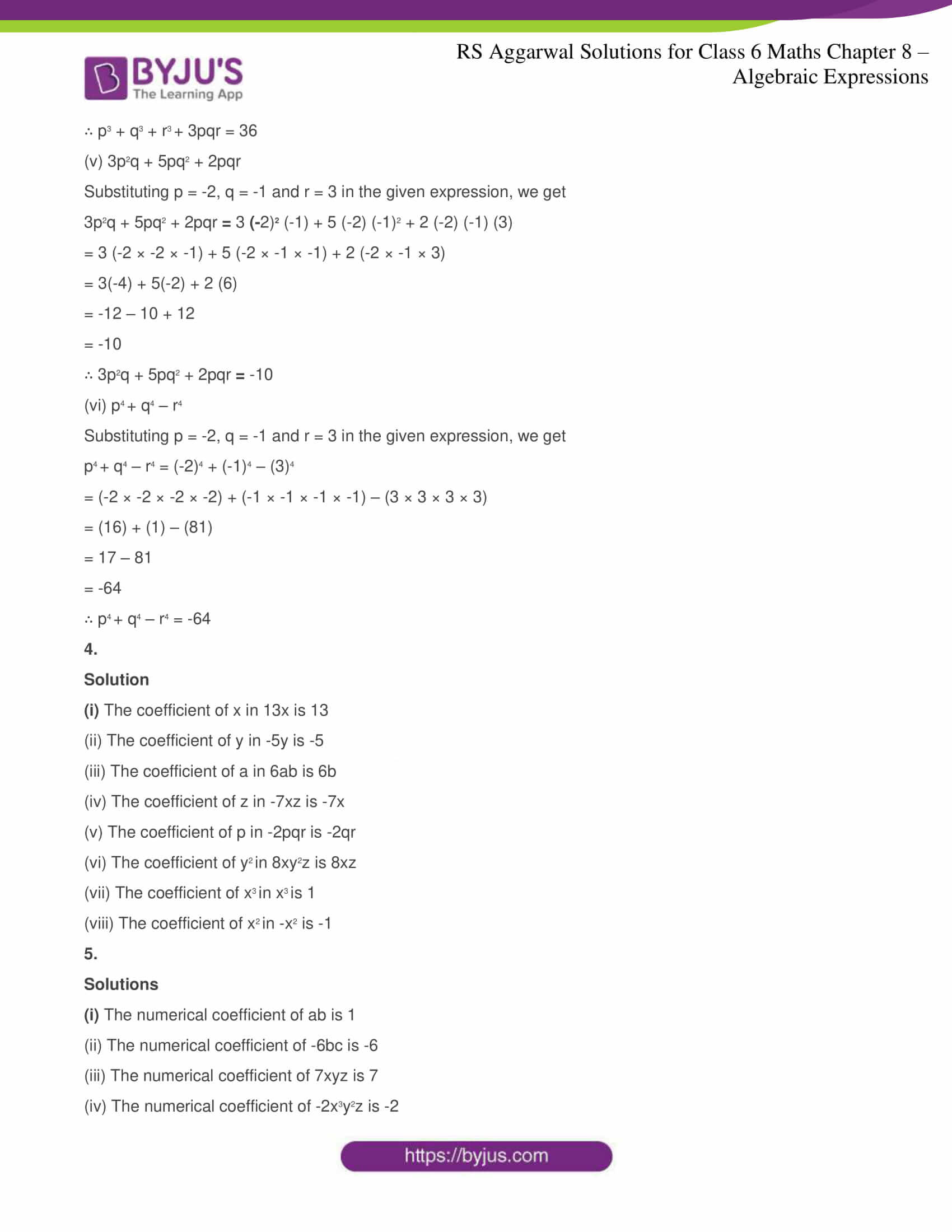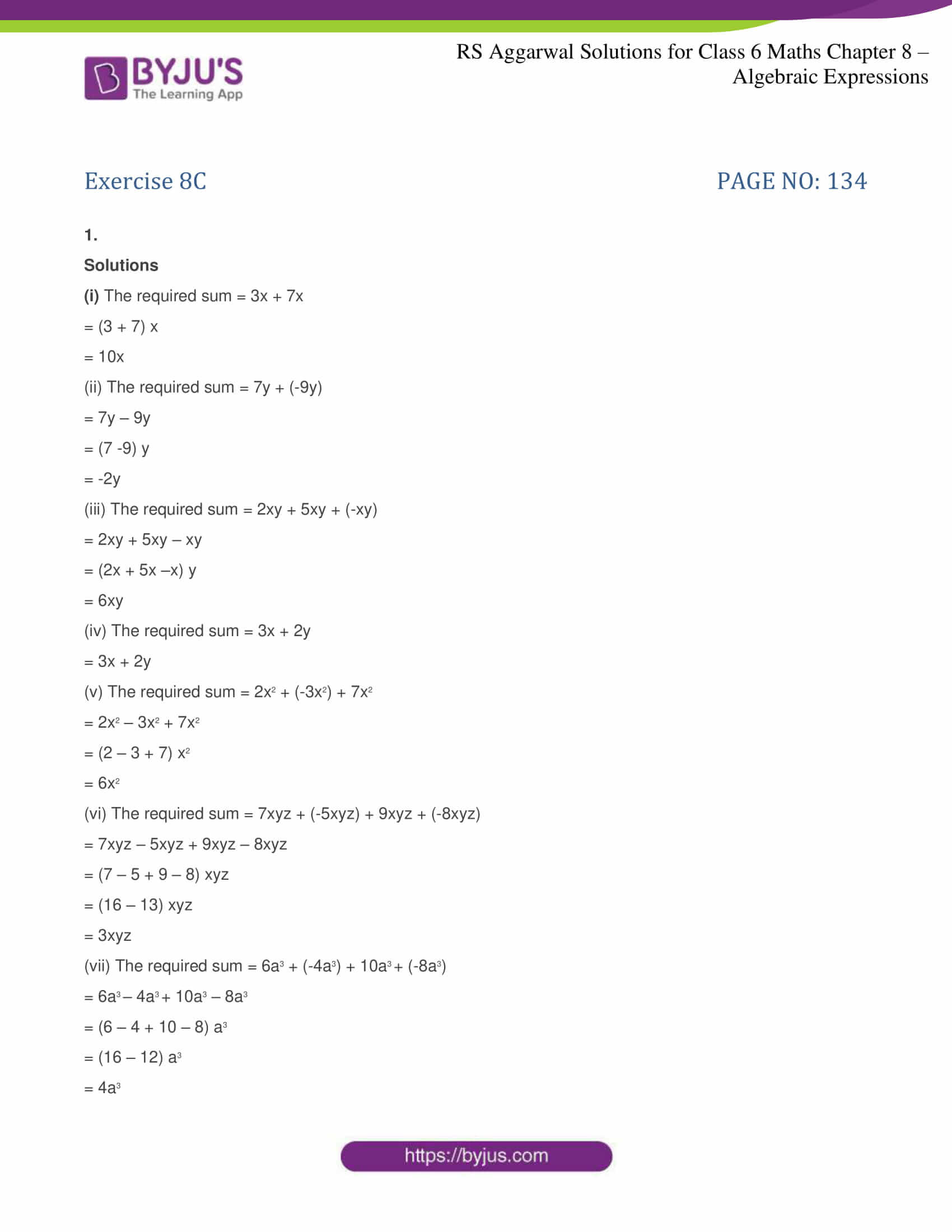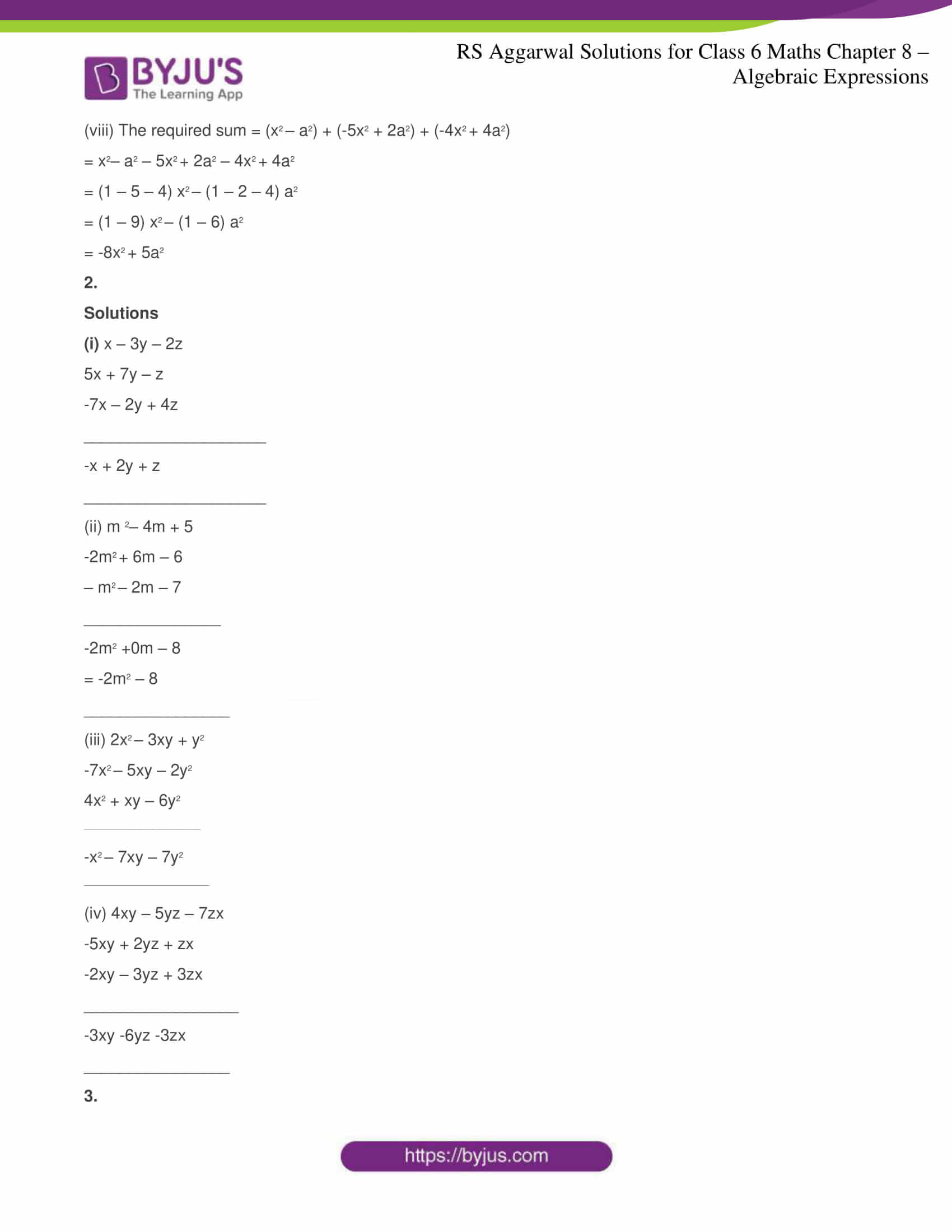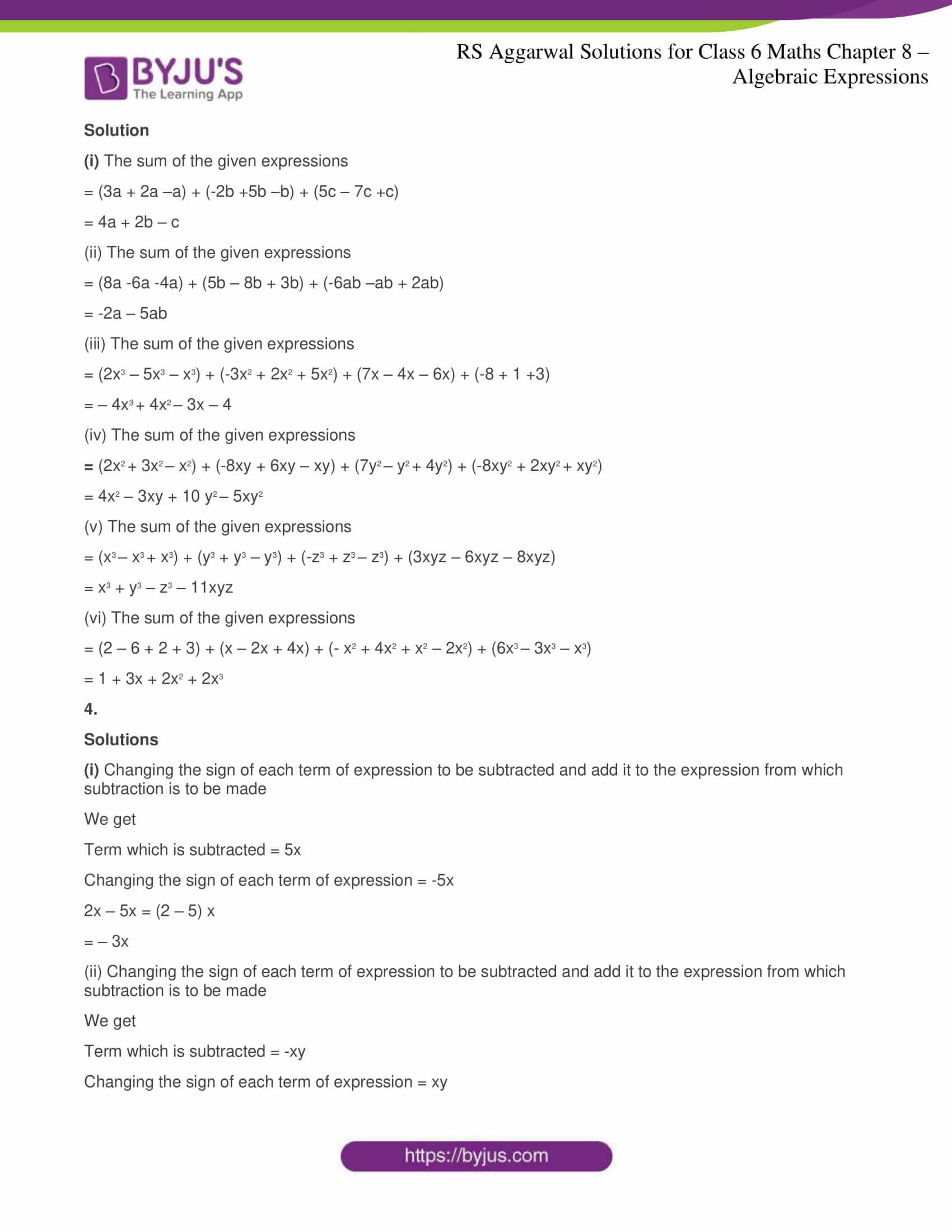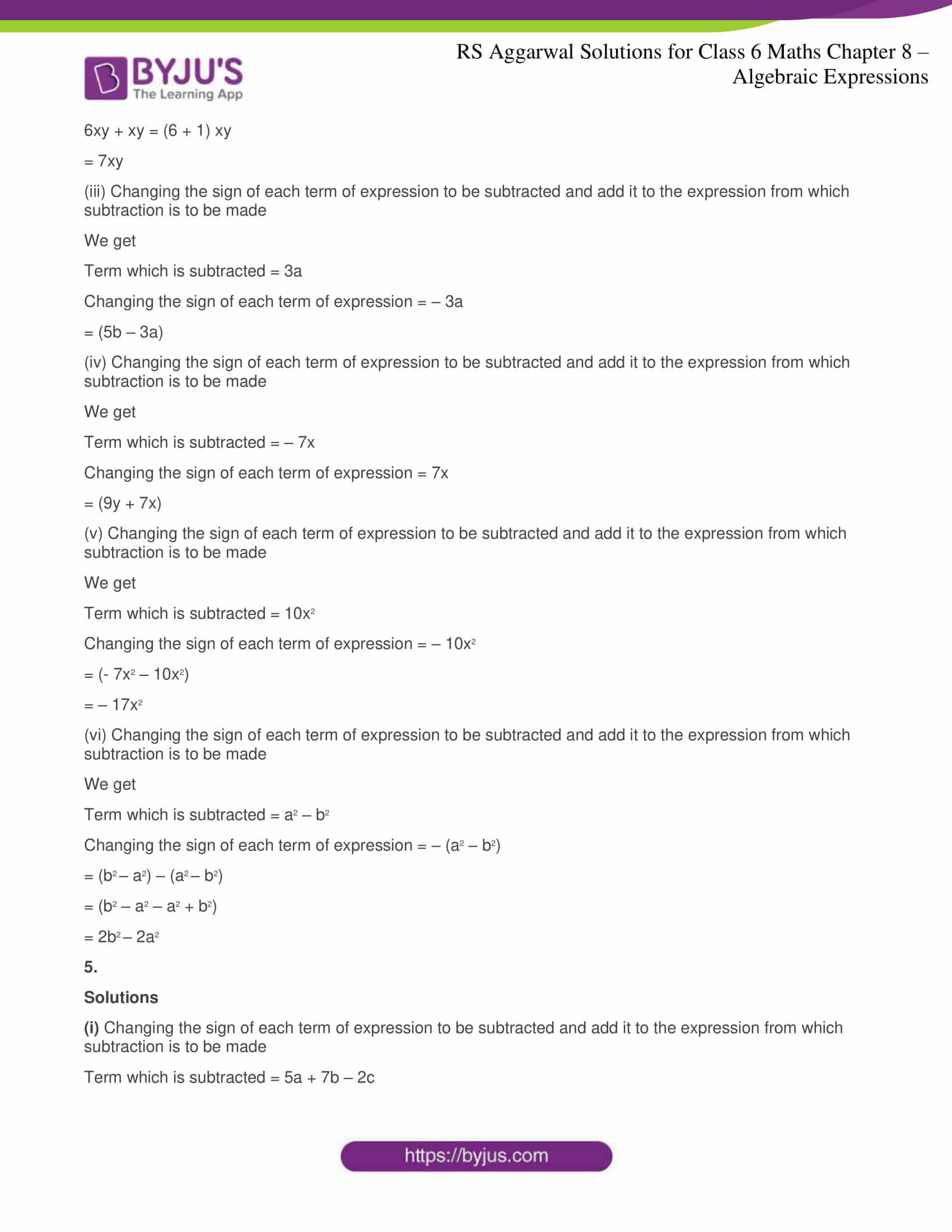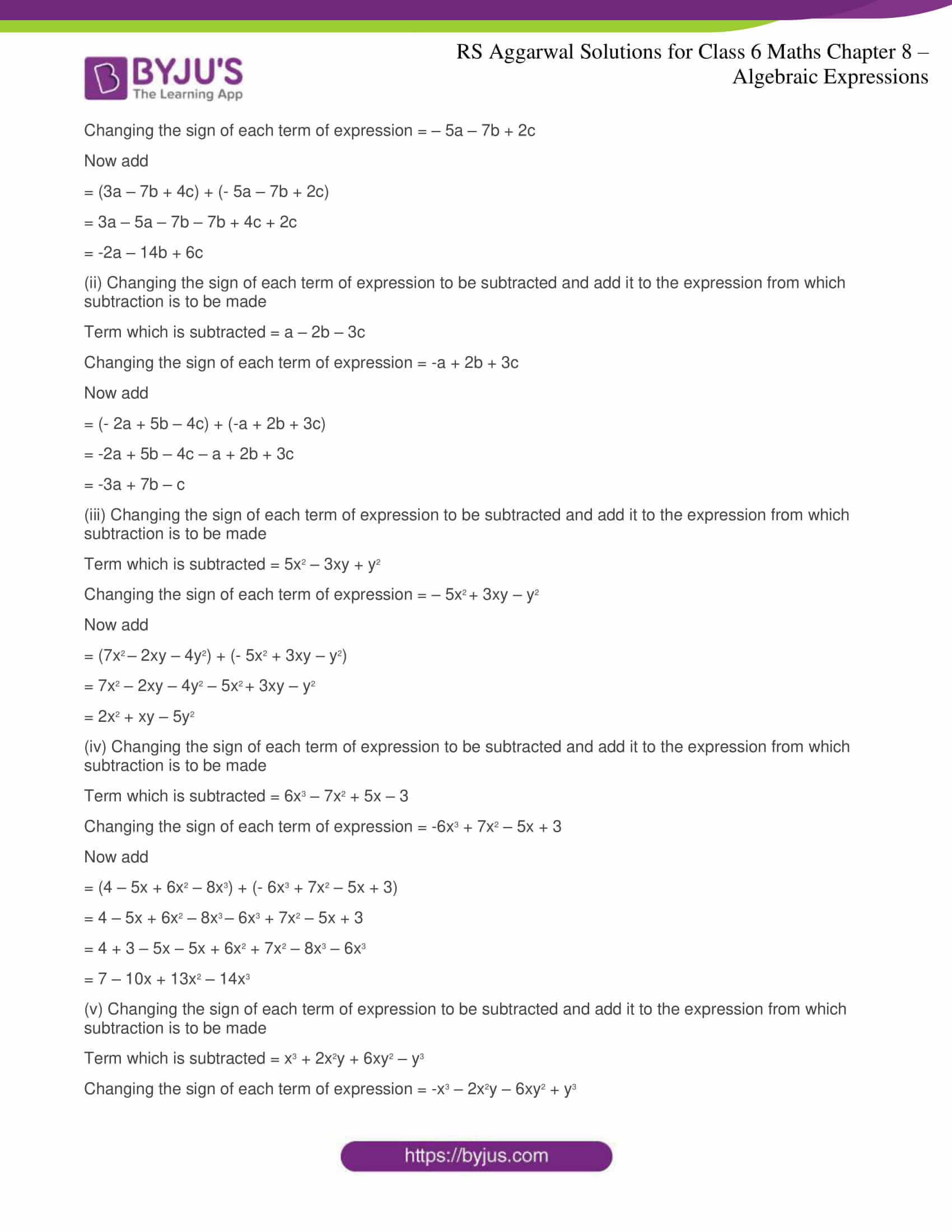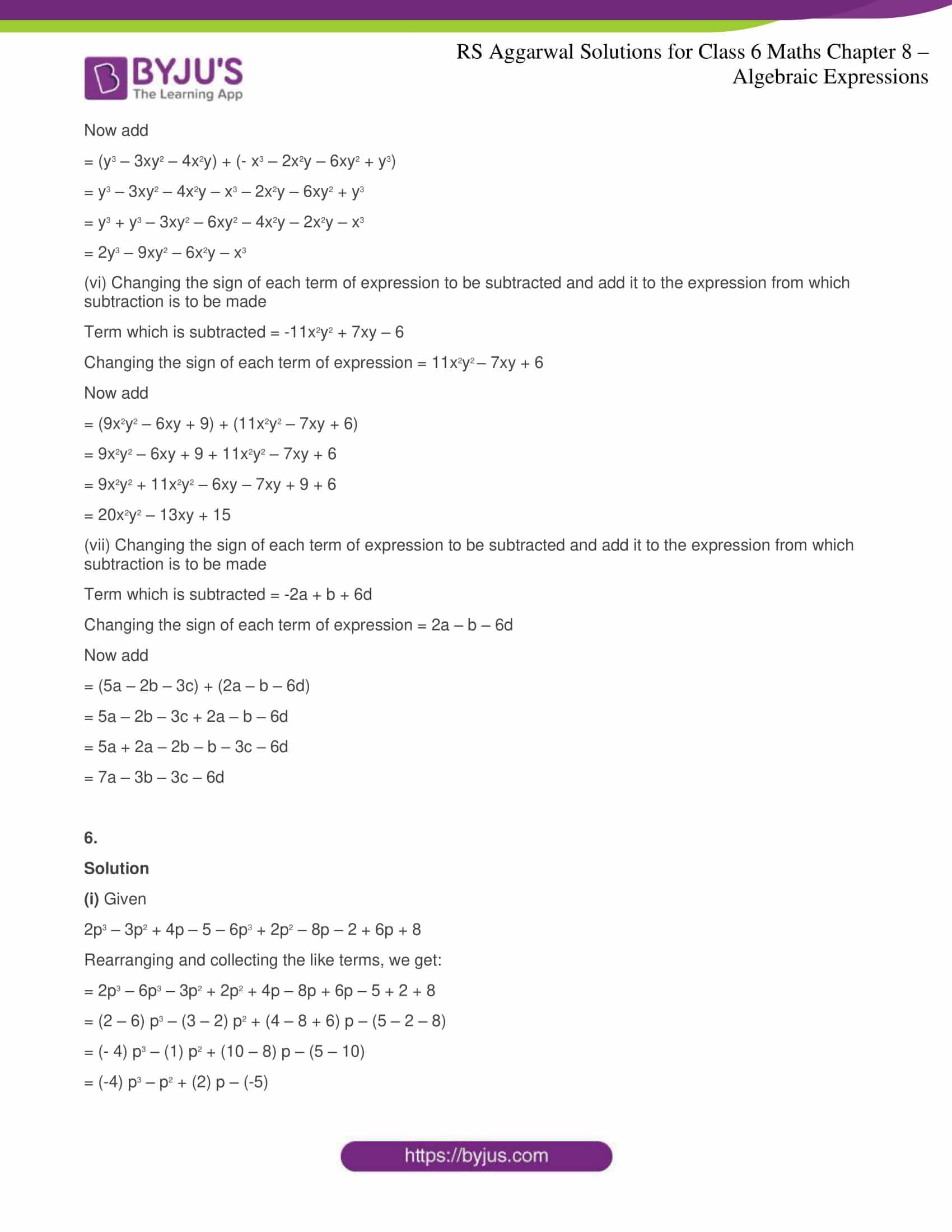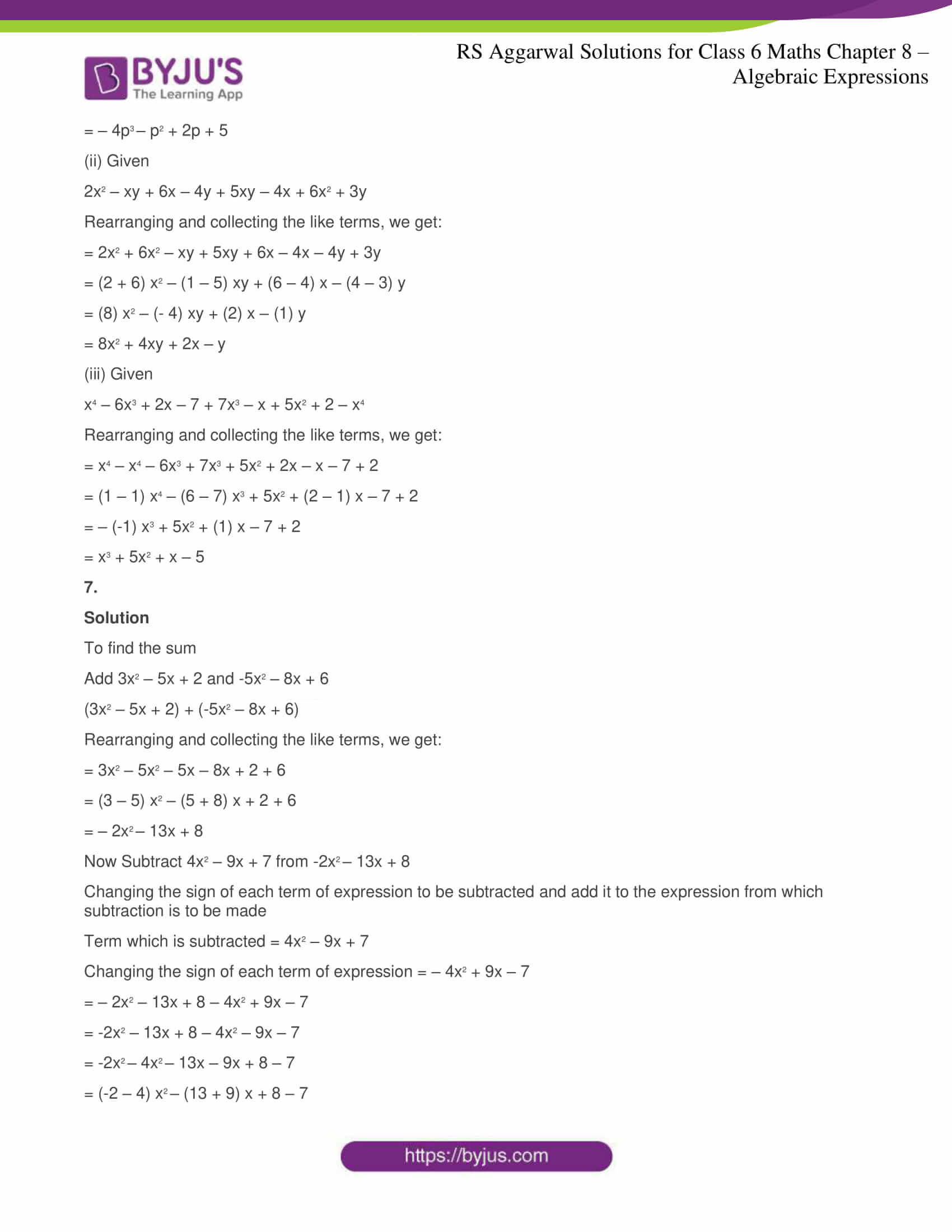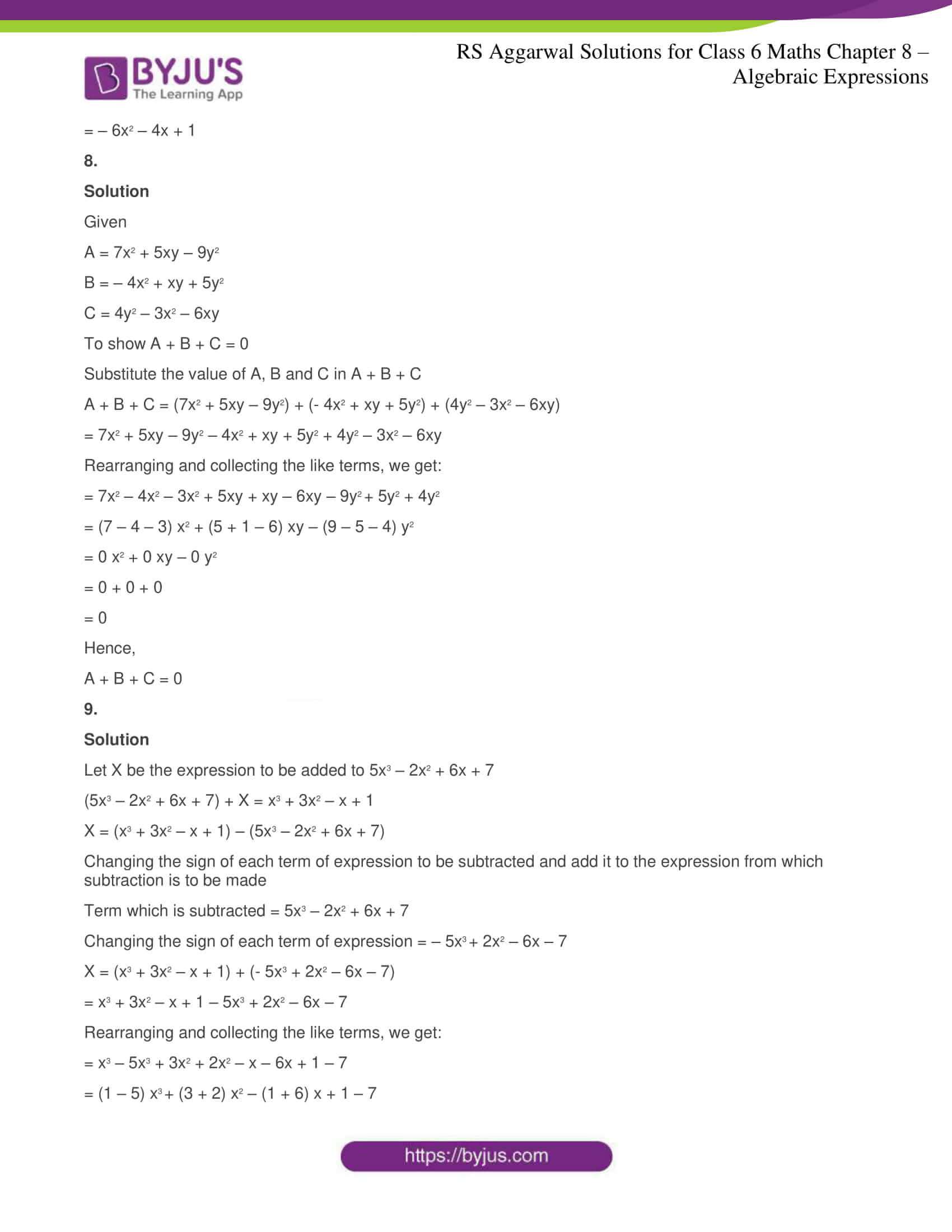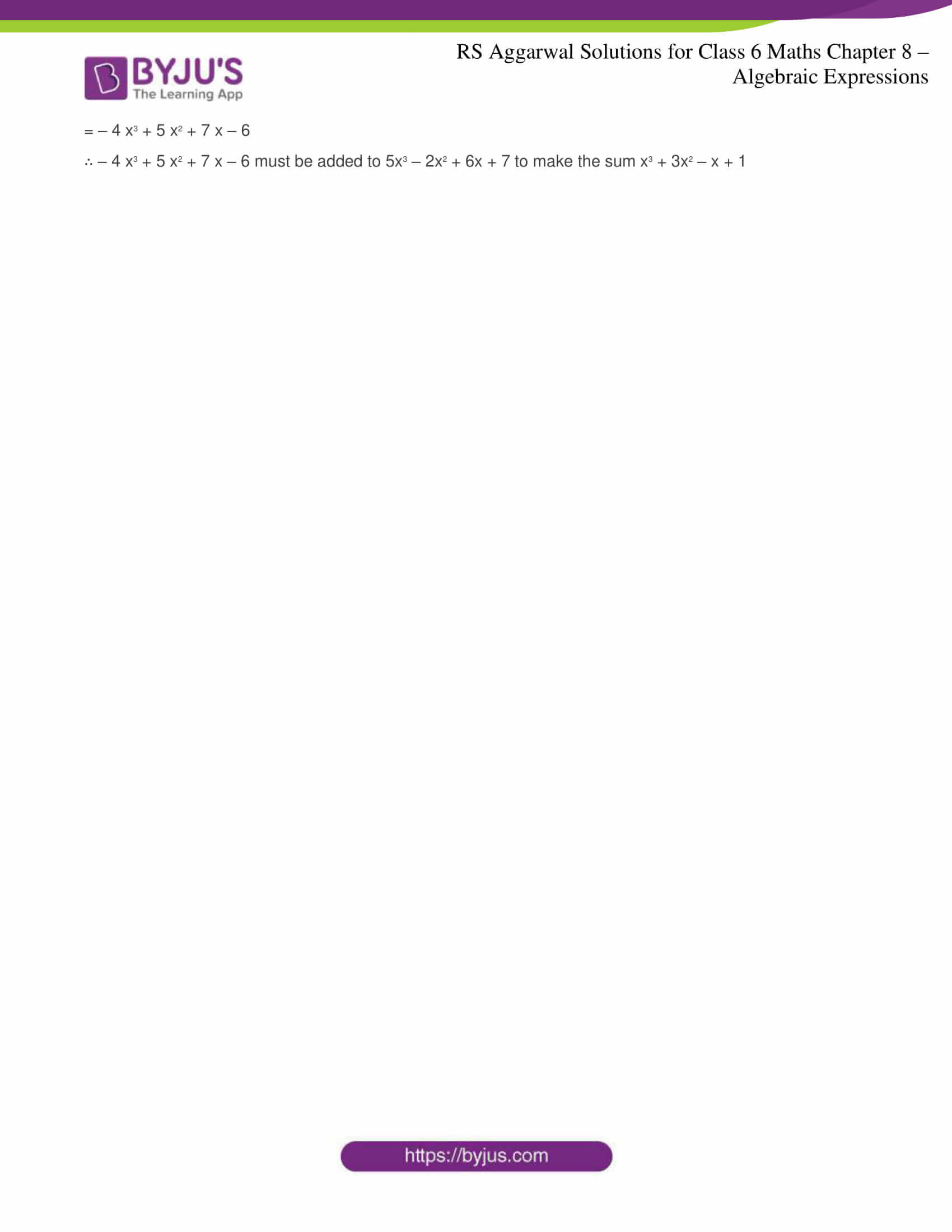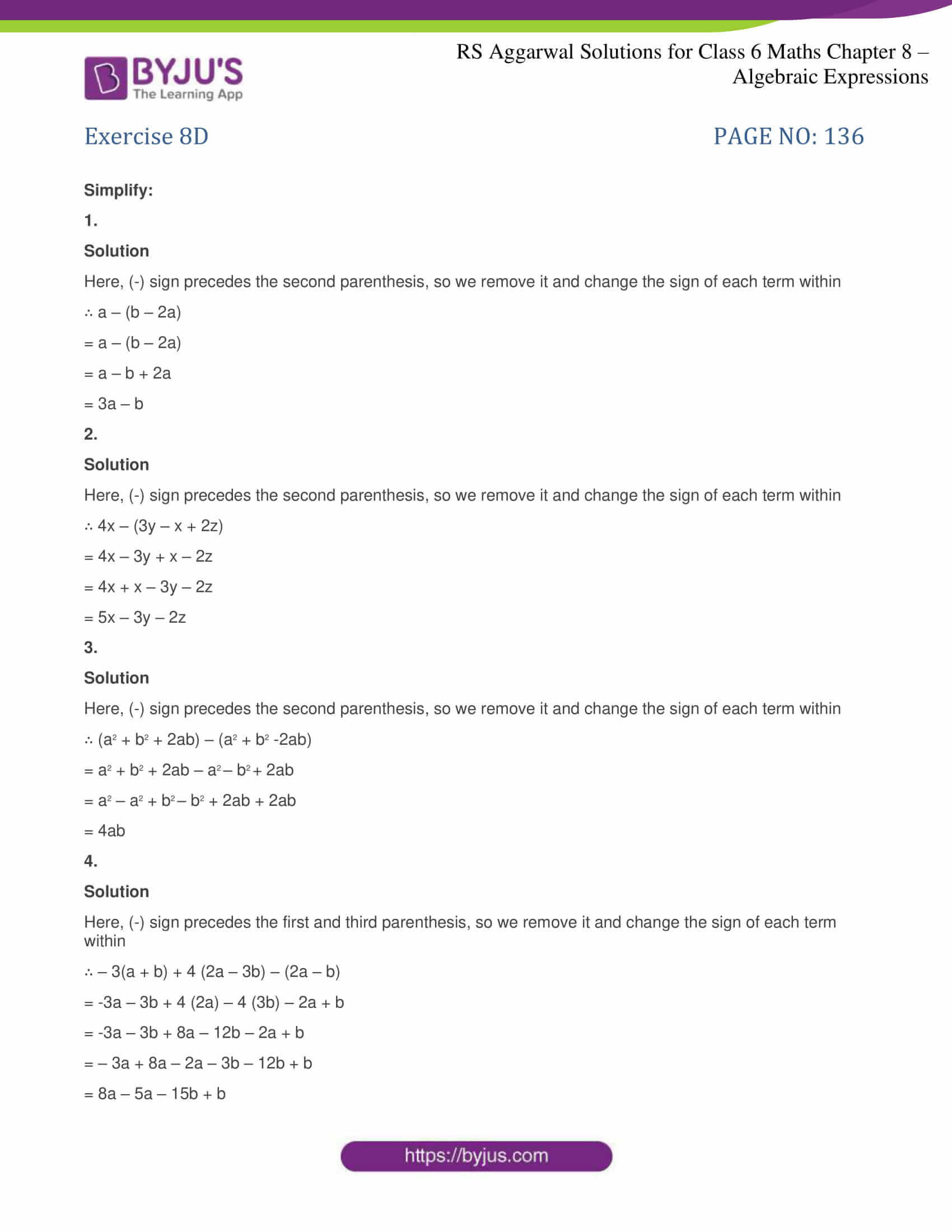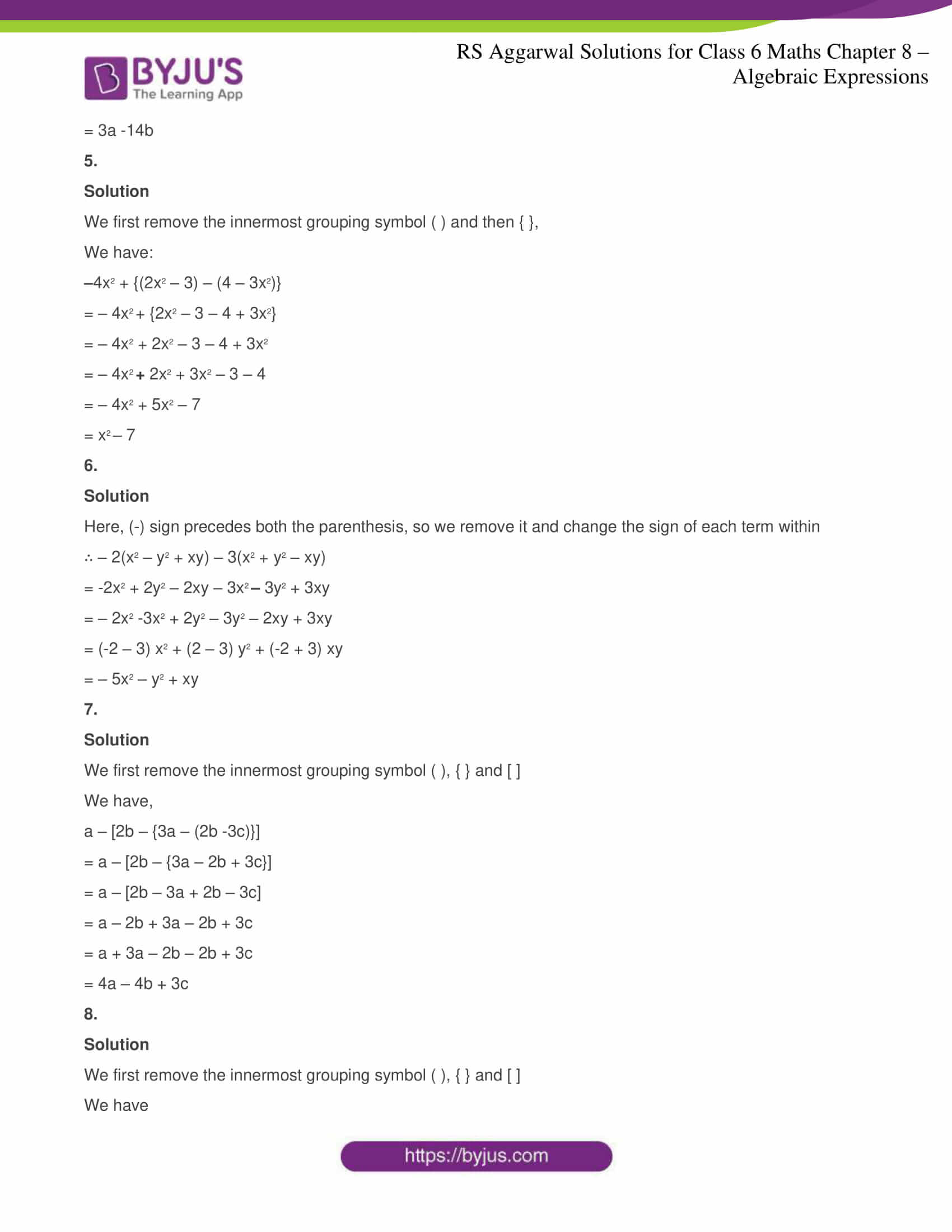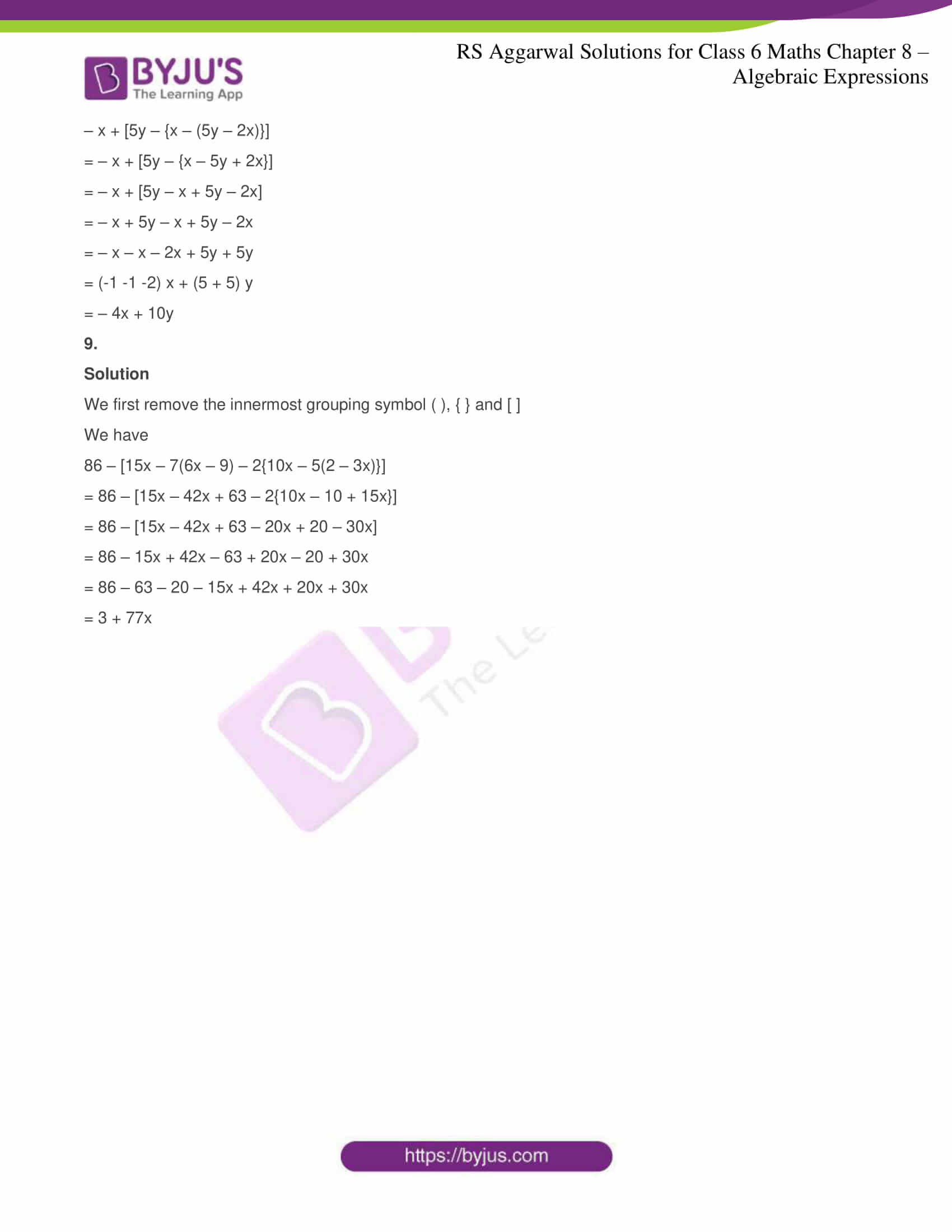### RS Aggarwal Solutions for Class 6 Maths Chapter 8 Algebraic Expressions

Chapter 8 – Algebraic Expressions consists of 4 exercises. RS Aggarwal Solutions have been solved in detail for each question in every exercise. Let’s have a glance at the topics included in this Chapter

• Operations on Literals and Numbers
• Algebraic Expressions
• Operations on Algebraic Expressions
• Use of grouping symbols

### Chapter Brief of RS Aggarwal Solutions for Class 6 Maths Chapter 8 – Algebraic Expressions

An expression having both variable and constant along with algebraic operations such as addition, subtraction, multiplication and division. For example, 6x – 4,

here x is the variable whose value is unknown, 6 is known as coefficient of x and 4 is the constant term having a definite value. There are five types of algebraic expressions.

(i) Monomials – expression having one term known as monomials

(ii) Binomials – expression having two terms known as binomials

(iii) Trinomials – expression having three terms known as trinomials